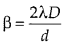Enlightened

# Question 17: NCERT Solutions for 12th Class Physics: Chapter 10-Wave Optics

• 0

Question 17: NCERT Solutions for 12th Class Physics: Chapter 10-Wave Optics

(a) In a single slit diffraction experiment, the width of the slit is made double the original width. How does this affect the size and intensity of the central diffraction band?
(b) In what way is diffraction from each slit related to the interference pattern in a double-slit experiment?
(c) When a tiny circular obstacle is placed in the path of light from a distant source, a bright spot is seen at the centre of the shadow of the obstacle. Explain why?
(d) Two students are separated by a 7 m partition wall in a room 10 m high. If both light and sound waves can bend around obstacles, how is it that the students are unable to see each other even though they can converse easily.
(e) Ray optics is based on the assumption that light travels in a straight line. Diffraction effects (observed when light propagates through small apertures/slits or around small obstacles) disprove this assumption. Yet the ray optics assumption is so commonly used in understanding location and several other properties of images in optical instruments. What is the justification?

Share

1. Solution:
(a) Linear width of central maximumOn doubling the slit width ‘d’, the size of central diffraction band is halved.
Because the width of central maximum is halved. Its area become 1/4 times and hence the intensity become 4 times the initial intensity.
(b) In double slit experiment, an interference pattern is observed by waves from two slits but as each slit provide a diffraction pattern of its own, thus the intensity of interference pattern in Young’s double slit experiment is modified by diffraction pattern of each slit.
(c) Waves from the distant source are diffracted by the edge of the circular obstacle and these waves superimpose constructively at the centre of obstacle’s shadow producing a bright spot.
(d) We know for diffraction to take place, size of the obstacle/aperture should be of the order of wavelength. Wavelength of sound waves is of the order of few meters that is why sound waves can bend through the aperture in partition wall but wavelength of light waves is of the order of micrometer, hence light waves can not bend through same big aperture. That is why the two students can hear each other but cannot see each other.(e) In optical instruments, the sizes of apertures are much larger as compared to wavelength of light. So the diffraction effects are negligibly small. Hence, the assumption that light travels in straight lines is used in the optical instruments.

Check the complete chapter with solutions.

NCERT Solutions for 12th Class Physics: Chapter 10-Wave Optics

• 0